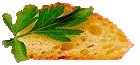Good Cooking since 1995

# Volume Conversions

It's all about volume and knowing at a moment of cooking what the amount is, wether it's in the US or Metric units of measure.

 U.S. Metric 1 tsp. (US) = 1/6 oz. 4.929 mL 1 tbsp. (US) = 0.5 oz. 14.79 mL 1 fluid oz. (US) = 1 oz. 29.57 mL 1 gill (US) = 4 oz. 118 mL 1 cup (US) = 8 oz. 236.6 mL 1 pint (US) = 16 oz. 473.2 mL 1 quart (US) = 32 oz. 946.3 mL 1 gallon (US) = 128 oz. 3.785 L 1 cubic inch = 0.5541 oz. 16.387 mL 1 tsp. (UK) = 1.2 tsp. 6.16 mL 1 dessert spoon (UK) = 2.4 tsp. 12.32 mL 1 tbsp. (UK) = 1.2 tbsp. 18.48 mL 1 fluid oz. (UK) = 0.96076 oz. 28.4 mL 1 gill (UK) = 1.2 gills 142 mL 1 breakfast cup (UK) = 1.2 cups 284 mL 1 pint (UK) = 1.2 pints 568 mL 1 quart (UK) = 1.2 quarts 1.136 L 1 gallon (UK) = 1.2 gallons 4.546 L 1 mLiter = 0.203 tsp. 1 mL 1 centiliter = 2.03 tsp. 10 mL 1 deciliter = 0.423 cup 100 mL 1 liter = 1.057 quarts 1000 mL 1 decaliter = 2.642 gallons 10000 mL 1 tsp. (metric) = 1.014 tsp. 5 mL 1 tbsp. (metric) = 3.04 tbsp. 15 mL 1 standard cup = 1.0567 cups 250 mL